Mathematical and Physical Journal
for High Schools
Issued by the MATFUND Foundation
 Already signed up? New to KöMaL?

# KöMaL Problems in Physics, October 2011

Show/hide problems of signs:## Problems with sign 'M'

Deadline expired on November 10, 2011.

M. 317. Cut sectors of different central anglesand of radius a=10 cm from A4 sheets of paper, and carefully fold and glue them such that they form right circular cones. The gained cones have different vertex angles, Let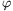be the half of the vertex angle. If they are dropped from a great height they will fall with constant speed. Measure how this terminal speed depends on the half of the vertex angle.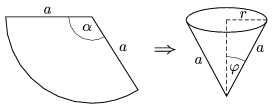(6 pont)

statistics## Problems with sign 'P'

Deadline expired on November 10, 2011.

P. 4370. A plane covers a distance of 2 km in 15 s when it flies downwind and in 20 s when it flies into the wind. Calculate the speed of the plane in km/h, and the speed of the wind.

(3 pont)

solution (in Hungarian), statistics

P. 4371. A pebble shot by a slingshot has an initial vertical, upward speed of 50 m/s. If there was no air drag

a) where would the pebble be after 7 seconds;

b) What would its speed be at that moment;

c) How much distance would it cover in 7 seconds?

(3 pont)

solution (in Hungarian), statistics

P. 4372. Two trolleys of mass 2 kg, lying along a line, are at rest on a horizontal tabletop. On one of them there is a spring as well as shown in the figure. The trolley on the left is given an initial speed of 5 m/s, and it collides with the other one on the right. What is the energy stored in the spring when it is the shortest?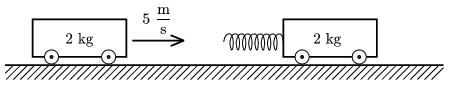(3 pont)

solution (in Hungarian), statistics

P. 4373. Bodies of mass m=0.5 kg, resting on a horizontal frictionless tabletop, are connected with an unstretched spring of length L=20 cm, and of spring constant D=16 N/m. The mass of the spring is negligible. At a certain moment the bodies are given an initial speed of v0=0.36 m/s, towards the wall on the right. The body at the right collides with the wall totally elastically.

a) What is the greatest compression of the spring during the motion?

b) After the collision with the wall how much time elapses until the moment when the bodies are the closest?

c) Will there be any other collision with the wall later? How will the system of bodies move if a long enough time elapses? d) What is the change in the linear momentum of the system after all collisions occurred?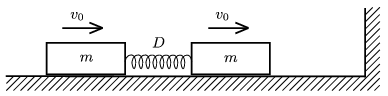(4 pont)

solution (in Hungarian), statistics

P. 4374. In a garden a spherical sprinkler is used at the end of the hose, from which the speed of the flowing water is the same to every direction. The water beam which is directed vertically upward reaches a height of h. Once the sprinkler is placed to the ground and at another time to a height of h. By what factor will the watered area be greater in the second case? (Neglect air resistance and consider the pressure in the sprinkler constant.)

(5 pont)

solution (in Hungarian), statistics

P. 4375. A sample of ideal gas of constant mass is taken through the cyclic process shown in the figure. Plot this cyclic process on the (p,T), and (E,V) diagrams instead of the (p,V). (E is the internal energy of the gas.)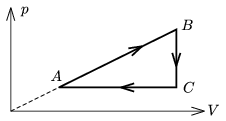(5 pont)

solution (in Hungarian), statistics

P. 4376. Two capacitors of capacitances C1=180 pF and C2=20 pF were connected in series, and their free terminals were connected to the terminals of a rechargeable battery which could supply a constant e.m.f. of U0=24 V. (The dielectric between the plates of both condensers was air.) Thus in both capacitors some energy was stored. Later the energy stored in the condensers was increased, with the same amount in both. This was done by filling totally the space between the plates of the capacitor of capacitance C2 by glass, the relative dielectric constant of which is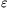r. The connection of the capacitors and the other capacitor of capacitance C1 were not changed.

a) What was the relative dielectric constantr of the glass which was used?

b) By what amount did the energy of the condensers increase? (The relative dielectric constant of air is 1.)

(5 pont)

solution (in Hungarian), statistics

P. 4377. A real coil was connected to an ohmic resistor of resistance 600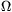in series. The voltages across the coil and the resistor are 200 V and 100 V, respectively. What are the inductance and the resistance of the coil if the root-mean-square value of the voltage supply is 230 V and its frequency is 50 Hz.

(4 pont)

solution (in Hungarian), statistics

P. 4378. In a particle accelerator a charged particle was accelerated to a speed of 80% of the speed of light. What will its speed be if it is further accelerated and its kinetic energy is doubled?

(4 pont)

solution (in Hungarian), statistics

P. 4379. The atomic mass number of Radium is A=226, its halflife is 1622 years. What is the activity of 1 g Radium? (Earlier this was the base of the unit of activity: 1 curie was the activity of 1 g radium.)

(3 pont)

solution (in Hungarian), statistics